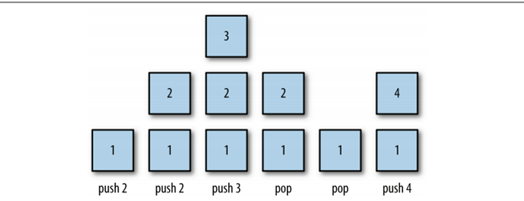function push(element){
this.dataStore[this.top++] = element;
}

function clear(){
this.top = 0
}

fact(5) //120

您可能感兴趣的文章:

javascript提供可操作的方法, 入栈 push, 出栈 pop，但是pop会移掉栈中的数据

function Stack(){
this.dataStore = []
this.top    = 0;
this.push   = push
this.pop    = pop
this.peek   = peek
this.length = length;
}

function pop(){
return this.dataStore[–this.top];
}

function isPalindrome(word) {
var s = new Stack()
for (var i = 0; i < word.length; i++) {
s.push(word[i])
}
var rword = “”;
while (s.length() > 0) {
rword += s.pop();
}
if (word == rword) {
return true;
} else {
return false;
}
}

function factorial(n) {
if (n === 0) {
return 1;
} else {
return n * factorial(n – 1);
}
}

5! = 5 * 4 * 3 * 2 * 1 = 120

isPalindrome(“aarra”) //false
isPalindrome(“aaraa”) //true

function length(){
return this.top
}function fact(n) {
var s = new Stack()
while (n > 1) {
//[5,4,3,2]
s.push(n–);
}
var product = 1;
while (s.length() > 0) {
product *= s.pop();
}
return product;
}

function peek(element){
return this.dataStore[this.top-1];
}